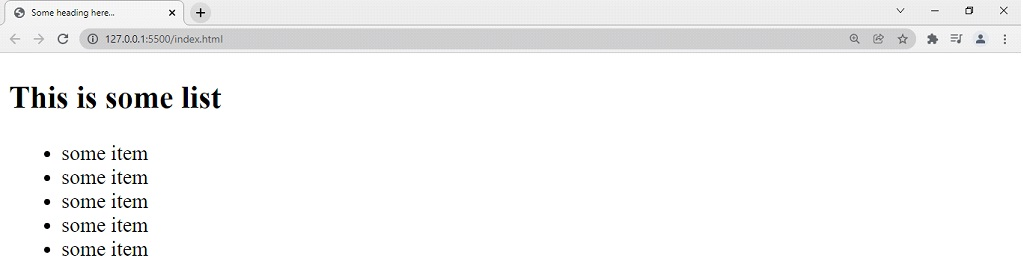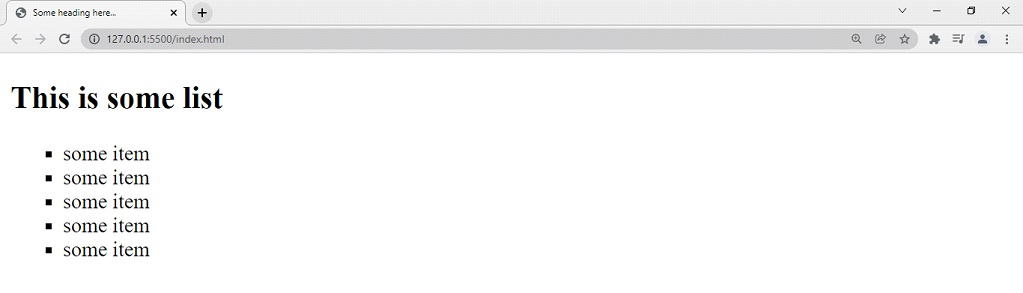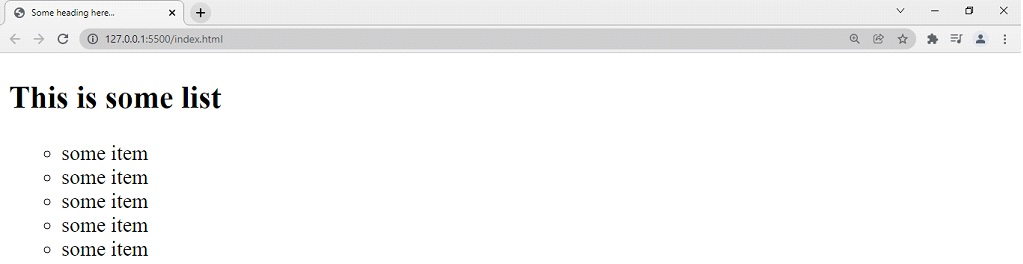# Unordered List HTML

Unordered List HTML

Similar to the ordered lists, the unordered lists also represent the content as a list. Just the thing is that there are no 1, 2, 3 or a, b, c, or such orders. Instead, we are going to use circles, squares, or discs here to represent the list of items. Simply, just like we have used the ol above, we are going to use ul here.

Have a look at the below example, to understand the unordered list →

<!DOCTYPE html>
<html lang=”en”>
<meta charset=”UTF-8″>
<meta http-equiv=”X-UA-Compatible” content=”IE=edge”>
<meta name=”viewport” content=”width=device-width, initial-scale=1.0″>
<title>Basic Web Page</title>
<body>
<ul>
<li>some item</li>
<li>some item</li>
<li>some item</li>
<li>some item</li>
<li>some item</li>
</ul>
</body>
</html>

If we try to open the file in the browser, the output that we get is something like this –As you can see here, the list items are seen as bullet points. Again, if you don’t want those bullet points(disc), but you want a square, you can change it. Again, we are going to use the type attribute to tell the browser that we want square bullets.

This is how you can do that →

<!DOCTYPE html>
<html lang=”en”>
<meta charset=”UTF-8″>
<meta http-equiv=”X-UA-Compatible” content=”IE=edge”>
<meta name=”viewport” content=”width=device-width, initial-scale=1.0″>
<body>
<h2>This is some list</h2>
<ul type=”square”>
<li>some item</li>
<li>some item</li>
<li>some item</li>
<li>some item</li>
<li>some item</li>
</ul>
</body>
</html>

So, now, if we try to open the file in the browser, the output is something like this –So, now you know that we were able to tell the browser to show the square bullets with the help of the type attribute. Similarly, we can also tell the browser to show the bullets as shallow circles, or discs, again just by telling it through the type attribute. The default thing is the disc. You can try the value as a disc. Let’s also try putting circles, to get the shallow circle as markers.

You can refer to the program below which demonstrates the circle markers in the unordered list →

<!DOCTYPE html>
<html lang=”en”>
<meta charset=”UTF-8″>
<meta http-equiv=”X-UA-Compatible” content=”IE=edge”>
<meta name=”viewport” content=”width=device-width, initial-scale=1.0″>
<body>
<h2>This is some list</h2>
<ul type=”circle”>
<li>some item</li>
<li>some item</li>
<li>some item</li>
<li>some item</li>
<li>some item</li>
</ul>
</body>
</html>

If we try opening the file in the browser, the output is something like this –So, now you can have some specific markers for the list items, according to the requirement.

Here are some different values available for the type attribute that we can use here…

 Value of the type attribute Marker description type =”disc” This is the default thing. type =”square” When the square value is given, the markers appear as squares. type =”circle” When the value circle is given, the markers appear as shallow circles.